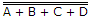# Electronics and Communication Engineering - Digital Electronics

1.

The counter which require maximum number of FF for a given mod counter is

 A. Ripple counter B. BCD counter C. Ring counter D. Programmed counter

Explanation:

No answer description available for this question. Let us discuss.

2.

Four inputs A, B, C, D are fed to a NOR gate. The output of NOR gate is fed to an inverter. The output of inverter is

 A. A + B + C + D B. A + B + C + D C. ABCD D. ABCD

Explanation:

Output == A + B + C + D.

3.

The content of which of the following determines the state of the CPU at the end of the execute cycle (when the interrupt is recognized)?

1. Program counter
2. Processor register
3. Certain status conditions
Select the correct answer using the codes given below :

 A. 1 and 2 B. 2 and 3 C. 1 and 3 D. 1, 2 and 3

Explanation:

It is the state at which interrupt is recognized program counter and certain status condition.

4.

In a gate output is Low if and only if all inputs are High. The gate is

 A. OR B. AND C. NOR D. NAND

Explanation:

No answer description available for this question. Let us discuss.

5.

With on-chip decoding, n address lines can access

 A. 2n memory locations B. 2n2 memory locations C. 2n memory locations D. n2 memory location